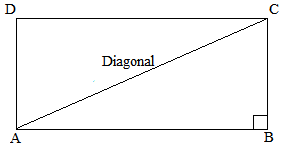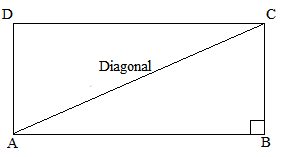# RD Sharma Class 6 ex 20.5 Solutions Chapter 20 Mensuration

In this chapter, we provide RD Sharma Class 6 ex 20.5 Solutions Chapter 20 Mensuration for English medium students, Which will very helpful for every student in their exams. Students can download the latest RD Sharma Class 6 ex 20.5 Solutions Chapter 20 Mensuration Maths pdf, Now you will get step by step solution to each question.

# Chapter 20: Mensuration Exercise 20.5

### Question: 1

The sides of a rectangle are in the ratio 5 : 4. If its perimeter is 72 cm, then its length is

(a) 40 cm

(b) 20 cm

(c) 30 cm

(d) 60 cm

### Solution:

(b) 20 cm

Explanation:

Let the sides of the rectangle be 5x and 4x. (Since, they are in the ratio 5 : 4)

Now, perimeter of rectangle = 2 (Length + Breadth)

72 = 2 (5x + 4x)

72 = 2 × 9x

72 = 18x

x = 4

Thus, the length of the rectangle = 5x = 5 × 4 = 20 cm

### Question: 2

The cost of fencing a rectangular field 34 m long and 18 m wide at As 2.25 per metre is

(a) Rs 243

(b) Rs 234

(c) Rs 240

(d) Rs 334

### Solution:

(b) Rs. 234

Explanation:

For fencing the rectangular field, we need to find the perimeter of the rectangle.

Length of the rectangle = 34 m

Breadth of the rectangle = 18 m

Perimeter of the rectangle = 2 (Length + Breadth) = 2 (34 + 18) m = 2 × 52 m = 104 m

Cost of fencing the field at the rate of Rs. 2.25 per meter = Rs. 104 × 2.25 = Rs. 234

### Question: 3

If the cost of fencing a rectangular field at Rs. 7.50 per metre is Rs. 600, and the length of the field is 24 m, then the breadth of the field is

(a) 8 m

(b) 18 m

(c) 24 m

(d) 16 m

### Solution:

(d) 16 m

Explanation:

Cost of fencing the rectangular field = Rs. 600

Rate of fencing the field = Rs. 7.50 per m

Therefore, perimeter of the field = Cost of fencing / Rate of fencing = 600 / 7.50 = 80 m

Now, length of the field = 24 m

Therefore, breadth of the field = Perimeter/2 – Length = 80/2- 24 = 16 m

### Question: 4

The cost of putting a fence around a square field at As 2.50 per metre is As 200. The length of each side of the field is

(a) 80 m

(b) 40 m

(c) 20 m

(d) None of these

### Solution:

(c) 20 m

Explanation:

Cost of fencing the square field = Rs. 200

Rate of fencing the field = Rs. 2.50

Now, perimeter of the square field = Cost of fencing / Rate of fencing = 200/2.50 = 80 m

Perimeter of square = 4 × Side of the square

Therefore, side of the square = Perimeter/4 = 80/4 = 20 m

### Question: 5

The length of a rectangle is three times of its width. If the length of the diagonal is 8√10 m, then the perimeter of the rectangle is

(a) 15√10 m

(b) 16√10 m

(c) 24√10 m

(d) 64 m

### Solution:

(d) 64 m

Explanation:

Let us consider a rectangle ABCD.

Also, let us assume that the width of the rectangle, i.e., BC be x m.It is given that the length is three times width of the rectangle.

Therefore, length of the rectangle, i.e., AB = 3x m

Now, AC is the diagonal of rectangle.

In right angled triangle ABC.

AC2 = AB2 + BC2

640 = 9x2 + x2

640 = 10x2

x2 = 640/10 = 64

x = 64 = 8 m

Thus, breadth of the rectangle = x = 8 m

Similarly, length of the rectangle = 3x = 3 × 8 = 24 m

Perimeter of the rectangle = 2 (Length + Breadth)

= 2 (24 + 8)

= 2 × 32 = 64 m

### Question: 6

If a diagonal of a rectangle is thrice its smaller side, then its length and breadth are in the ratio

(a) 3:1

(b) √3:1

(c) √2:1

(d) 2√2:1

### Solution:

(d) 22 : 1

Explanation:

Let us assume that the length of the smaller side of the rectangle, i.e., BC be x and length of the larger side , i.e., AB be y.

It is given that the length of the diagonal is three times that of the smaller side.

Therefore, diagonal = 3x = ACNow, applying Pythagoras theorem, we get:

(Diagonal)2 = (Smaller side)2 + (Larger side)2

(AC)2 = (AB)2 + (BC)2

(3x)2 = (x)2 + (y)2

9x2 = x2 + y2

8x2 = y2

Now, taking square roots of both sides, we get:

22 x = y

or, y / x = 22 / 1

Thus, the ratio of the larger side to the smaller side = 22 : 1

### Question: 7

The ratio of the areas of two squares, one having its diagonal double than the other, is

(a) 1 : 2

(b) 2:3

(c) 3 : 1

(d) 4 : 1

### Solution:

(d) 4 : 1

Explanation:

Let the two squares be ABCD and PQRS. Further, the diagonal of square PQRS is twice the diagonal of square ABCD

PR = 2 AC

Now, area of the square = (diagonal)2/2

Area of PQRS = (PR)2)/2

Similarly, area of ABCD = (AC)2/2

According to the question:

If AC = x units, then, PR = 2x units

Thus, the ratio of the areas of squares PQRS and ABCD = 4:1

### Question: 8

If the ratio of areas of two squares is 225:256, then the ratio of their perimeters is

(a) 225 : 256

(b) 256 : 225

(c) 15:16

(d) 16 : 15

### Solution:

(c) 15 : 16

Explanation:

Let the two squares be ABCD and PQRS.

Further, let the lengths of each side of ABCD and PQRS be x and y, respectively.

Therefore Area of sq. ABCD / Area of sq. PQRS = x2 / y2

⟹ x2/y= 225 / 256

Taking square roots on both sides, we get:

x/y = 15/16

Now, the ratio of their perimeters:

Perimeter of sq. ABCD / Perimeter of sq. PQRS

= 4 × side of sq. ABCD / 4 × Side of sq. PQRS = 4x / 4y

Perimeter of sq. ABCD / Perimeter of sq. PQRS = x y

Perimeter of sq. ABCD / Perimeter of sq. PQRS = 15 / 16

Thus, the ratio of their perimeters = 15:16

### Question: 9

If the sides of a square are halved, then its area

(a) remains same

(b) becomes half

(c) becomes one fourth

(d) becomes double

### Solution:

(c) becomes one fourth

Explanation:

Let the side of the square be x.

Then, area = (Side × Side) = (x × x) = x2

If the sides are halved, new side = x/2

Now, new area = (x/2)2

= (x2)/4

It is clearly visible that the area has become one-fourth of its previous value.

### Question: 10

A rectangular carpet has area 120 m2 and perimeter 46 metres. The length of its diagonal is

(a) 15 m

(b) 16 m

(c) 17 m

(d) 20 m

### Solution:

(c) 17 m

Explanation:

Area of the rectangle = 120 m2

Perimeter = 46 m

Let the sides of the rectangle be l and b.

Therefore

Area = lb = 120 m2 …(1)

Perimeter = 2 (l + b) = 46

Or, (l + b) = 46 / 2 =23 m …(2)

Now, length of the diagonal of the rectangle = l2 + b2

So, we first find the value of (l2 + b2)

Using identity:

(l2 + b2) = (l + b)2 – 2 (lb) [From (1) and (2)]

Therefore

(l2 + b2) = (23)2 – 2 (120)

= 529 – 240 = 289

Thus, length of the diagonal of the rectangle = l2 + b2 = 289 = 17 m

### Question: 11

If the ratio between the length and the perimeter of a rectangular plot is 1: 3, then the ratio between the length and breadth of the plot is

(a) 1 : 2

(b) 2 : 1

(c) 3 : 2

(d) 2 : 3

### Solution:

(b) 2 : 1

Explanation:

It is given that Length of rectangle / Perimeter of rectangle = 1 / 3

⟹ l/(2l + 2b) = 1 / 3

After cross multiplying, we get:

3l = 2l + 2b

⟹ l = 2b

⟹ l / b= 2 / 1

Thus, the ratio of the length and the breadth is 2: 1.

### Question: 12

If the length of the diagonal of a square is 20 cm, then its perimeter is

(a) 10√2 cm

(b) 40 cm

(c) 40√2 cm

(d) 200 cm

### Solution:

(c) 40√2  cm

Explanation:

Length of diagonal = 20 cm

All Chapter RD Sharma Solutions For Class 6 Maths

I think you got complete solutions for this chapter. If You have any queries regarding this chapter, please comment on the below section our subject teacher will answer you. We tried our best to give complete solutions so you got good